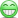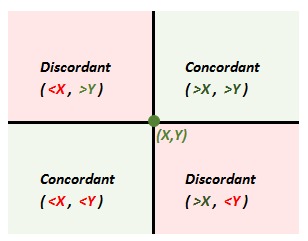# Concordant and discordant values

#### Kavita.bhangdia

##### Active Member
Hi David,
Please can you explain me concordant and discordant values in English..? I mean how to look for those values.. In the meisener reading you have represented it mathematically and I am not able to understand that.

Thanks
Kavita

#### QuantMan2318

##### Well-Known Member
Subscriber
You are referring to the Kendall's tau?
If so, then do the Spearman Rank correlation's first step of Ranking the Returns, once we Rank the Returns of a set of Assets say A and B , the values of the Rank constitute the x and y values

Look carefully at the magnitude ( the values )of the Rank, we get pairs of numbers for each pair of Assets. The example in Meissner uses 5 pairs of Returns corresponding to 5 years. According to Combinatorics, we can choose two pairs in exactly 5C2 ways which is 10 ways, so write down the pairs of numbers, this is important as this is the value of t and t* that I will explain shortly

To make it clearer, look at the Table below: (The same as Meissner)

There we see the following pairs of Ranks
(1,4) (2,5) (3,3) (4,1) (5,2), we can evaluate two out of these five pairs of observations in 10 ways

(1,4) (2,5); (2,5)(3,3); (3,3)(4,1); (4,1)(5,2);
(1,4) )(3,3); (2,5)(4,1); (3,3)(5,2);
(1,4)(4,1); (2,5)(5,2);
(1,4)(5,2);

Out of these pairs, look at each of the ranks in the pair, concordant pairs are those where the x value is more than the y value in both the set of two numbers in the pair (or x value is less than the y value), these are (1,4)(2,5) and (4,1)(5,2) which makes it two

Discordant pairs are those wherein either the x is greater than y in one set and y is greater than x in the other set or vice versa. these are (1,4)(4,1); (1,4)(5,2); (2,5)(4,1); (2,5)(5,2); which makes it four

whenever a set in a pair has x and y as equal they are neither, these are (1,4)(3,3); (2,5)(3,3); (3,3)(4,1); (3,3)(5,2);

In plain English, concordant pairs are those that have the sizes of their x and y values in concordance (in agreement) with each other, discordant pairs are those that have the sizes of their x and y values to be in disagreement with each other ( Definition mine)

#### Attachments

• Workbook1.xlsx
36.3 KB · Views: 30
Last edited:

#### David Harper CFA FRM

##### David Harper CFA FRM
Staff member
Subscriber
Awesome @QuantMan2318 ! ... except please notice that Meissner's text contains a mistake, see https://forum.bionicturtle.com/threads/kendalls-t-which-is-correct.9447/
.... his example contains 2 concordant pairs and eight (8) discordant pairs; e.g., (1,4)(3,3) is discordant.

I was thinking about this visually. If (X,Y) is the first pair represented by the intersection, then concordant pairs fall into the green regions and discordant fall into the red regions, relatively speaking:I hope that's interesting!

Last edited:

#### QuantMan2318

##### Well-Known Member
Subscriber
Thanks @David Harper CFA FRM for putting me in the picture and I am sorry, I missed Kenji san's post.

@Kenji Thanks very much for asking these questions, or else, I wouldn't have known the correct stuff

Last edited:

#### David Harper CFA FRM

##### David Harper CFA FRM
Staff member
Subscriber
Hi @QuantMan2318 FYI, I couldn't resist adding "neither" bars to the graphic (see below), I dressed this up further to include in the next version of our notes.#### Kavita.bhangdia

##### Active Member
Hi ,
One quick question regarding Kendalls tau.. Do we have to arrange one leg with ranks 1 to 5(assuming n=5).

And then get concordat and discordant values.. ? Thanks

Kavita

#### David Harper CFA FRM

##### David Harper CFA FRM
Staff member
Subscriber
Hi @Kavita.bhangdia No, the arrangement of the pairs is irrelevant. Take QuantMan's example above (which is Meissner's example); with 5 pairs there 5*4/2 = 10 comparisons and the order does not matter. It might be a little easier to count them if the X are ordered, but it won't matter on the result; e.g.,

(1,4) <- 1 C and 3 D; i.e., comparing to all other four with greater Xs, so any lesser Y is discordant
(2,5) <- 0 C and 3 D; i.e., comparing to three with greater Xs, but all have lesser Ys so all three discordant
(3,3) <- 0 C and 2 D; i.e., comparing to two with greater Xs but both have lesser Ys so both discordant
(4,1) <- 1 C and 0 D; i.e., comparing only to (5,5) which has both X and Y greater, so concordant. Total concordant = 2 and total discordant = 8, such that Kendall's tau = (2-8)/10 = -0.60. I hope that helps!
(5,2)

#### JeffVanCott

##### New Member
David have you seen the 2018 Schweser Part 2 Mock Exam 1 question 76? If not, here are the details and I would like you to let me know what Kendall correlation coefficient you receive.

Year X Y
2010 -5% 5%
2011 15% -10%
2012 -10% -5%
2013 25% 20%
2014 8% 35%

I get 0.2, but Schweser implies it is -0.2. Would you be able to let me know what you get? Thank you.

#### emilioalzamora1

##### Well-Known Member
Hi @JeffVanCott,

Kendall's Tau should be + 0.2 while Spearman's Rank is -0.2.

Having a rank for X and Y of:

X Y
4 3
3 5
5 4
1 2
3 1

Spearman:

d^2 (squared differences in ranks)

1
9
1
1
4

To yield the sum of 16

Inserting this in Spearman's formula having 5 returns: 1 - { 6* 16 / [ 5*(5^2 - 1) ] = -0.2

For Kendall you have the right result anyway: 0.2.

Concordant pairs:
3
1
3
3
2

>>> sum which is 12/2 = 6 concordant pairs

Discordant pairs
1
3
1
1
2

>>> sum which is (8)/2 = 4 concordant pairs

#### JeffVanCott

##### New Member
Thank you. I was shocked when I saw they had it incorrect and no adjustment to their practice exam (with one week away), so wanted to make sure I was calculating it correctly.

Staff member
Subscriber

#### emilioalzamora1

##### Well-Known Member
Indeed @David Harper CFA FRM,

Apologies. I subtracted 1 from 0.8 but the formula is 1 - (the term 6*16/5*(5^2 - 1) to yield positive 0.2 for Spearman.